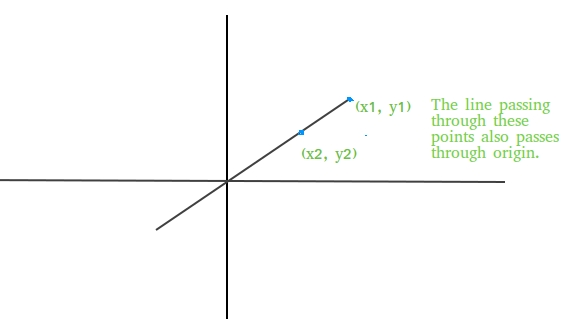# Check if a line passes through the origin

Given two coordinates of a line as (x1, y1) and (x2, y2), find if the line passing through these points also passes through origin or not.Examples:

```Input : (x1, y1) = (10, 0)
(x2, y2) = (20, 0)
Output : Yes
The line passing through these points
clearly passes through the origin as
the line is x axis.

Input : (x1, y1) = (1, 28)
(x2, y2) = (2, 56)
Output : Yes
```

## Recommended: Please try your approach on {IDE} first, before moving on to the solution.

Approach: Equation of a line passing through two points (x1, y1) and (x2, y2) is given by
y-y1 = ((y2-y1) / (x2-x1))(x-x1) + c
If line is also passing through origin, then c=0, so equation of line becomes
y-y1 = ((y2-y1) / (x2-x1))(x-x1)
Keeping x=0, y=0 in the above equation we get,
x1(y2-y1) = y1(x2-x1)
So above equation must be satisfied if any line passing through two coordinates (x1, y1) and (x2, y2) also passes through origin (0, 0).

## C++

 `/* C++ program to find if line passing through ` `   ``two coordinates also passes through origin  ` `   ``or not */` `#include ` `using` `namespace` `std; ` ` `  `bool` `checkOrigin(``int` `x1, ``int` `y1, ``int` `x2, ``int` `y2) ` `{ ` `   ``return` `(x1 * (y2 - y1) == y1 * (x2 - x1)); ` `} ` ` `  `// Driver code ` `int` `main() ` `{ ` `    ``if` `(checkOrigin(1, 28, 2, 56) == ``true``) ` `      ``cout << ``"Yes"``; ` `    ``else` `      ``cout << ``"No"``; ` `    ``return` `0; ` `} `

## Java

 `// Java program to find if line passing through ` `// two coordinates also passes through origin  ` `// or not  ` `import` `java.io.*; ` ` `  `class` `GFG { ` `     `  `    ``static` `boolean` `checkOrigin(``int` `x1, ``int` `y1,  ` `                                       ``int` `x2, ``int` `y2) ` `    ``{ ` `        ``return` `(x1 * (y2 - y1) == y1 * (x2 - x1)); ` `    ``} ` ` `  `    ``// Driver code ` `    ``public` `static` `void` `main (String[] args)  ` `    ``{ ` `        ``if` `(checkOrigin(``1``, ``28``, ``2``, ``56``) == ``true``) ` `            ``System.out.println(``"Yes"``); ` `        ``else` `            ``System.out.println(``"No"``); ` `    ``} ` `} ` ` `  `// This code is contributed by Ajit. `

## Python3

 `# Python program to find if line ` `# passing through two coordinates  ` `# also passes through origin or not ` ` `  `def` `checkOrigin(x1, y1, x2, y2): ` `    ``return` `(x1 ``*` `(y2 ``-` `y1) ``=``=` `y1 ``*` `(x2 ``-` `x1)) ` `     `  `# Driver code ` `if` `(checkOrigin(``1``, ``28``, ``2``, ``56``) ``=``=` `True``): ` `    ``print``(``"Yes"``) ` `else``: ` `    ``print``(``"No"``) ` `     `  `# This code is contributed ` `# by Anant Agarwal. `

## C#

 `// C# program to find if line passing through ` `// two coordinates also passes through origin ` `// or not ` `using` `System; ` ` `  `class` `GFG { ` ` `  `    ``static` `bool` `checkOrigin(``int` `x1, ``int` `y1, ` `                            ``int` `x2, ``int` `y2) ` `    ``{ ` `        ``return` `(x1 * (y2 - y1) == y1 * (x2 - x1)); ` `    ``} ` ` `  `    ``// Driver code ` `    ``public` `static` `void` `Main() ` `    ``{ ` `        ``if` `(checkOrigin(1, 28, 2, 56) == ``true``) ` `            ``Console.WriteLine(``"Yes"``); ` `        ``else` `            ``Console.WriteLine(``"No"``); ` `    ``} ` `} ` ` `  `// This code is contributed by vt_m. `

## PHP

 ` `

Output:

```Yes
```

Attention reader! Don’t stop learning now. Get hold of all the important DSA concepts with the DSA Self Paced Course at a student-friendly price and become industry ready.

My Personal Notes arrow_drop_upCheck out this Author's contributed articles.

If you like GeeksforGeeks and would like to contribute, you can also write an article using contribute.geeksforgeeks.org or mail your article to contribute@geeksforgeeks.org. See your article appearing on the GeeksforGeeks main page and help other Geeks.

Please Improve this article if you find anything incorrect by clicking on the "Improve Article" button below.

Improved By : jit_t

Article Tags :
Practice Tags :

1

Please write to us at contribute@geeksforgeeks.org to report any issue with the above content.